• 184
•
•
•
•
•
•
•
184
Shares

# DIFFERENCE BETWEEN RESISTANCE AND RESISTIVITY

In this article, we will discuss about the basic difference between the resistance and resistivity in a organised way. You will understand the underlying difference very clearly after going through this article. So stay tuned with us till end.

## RESISTANCE : BASIC

Resistance means to resist something which are going ahead. In any electrical conductor resistance is the property of the conductor due to which it resist the flow of electrons.

When electrons flow inside any conductor in the influence of electric field, it rushes towards the opposite direction of the electric field.

When it moves towards the positive end of the conductor it get collide with the atoms and molecules of the conductor due to which it’s velocity gets reduced. This type of obstructions caused due to the collision is called resistance.

Resistance is also defined as the ratio of potential difference to the current flowing.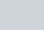It’s SI unit is ohm and denoted by Greek letter Omega (Ω).

Resistance of any conductor depends on the many factors such as

•  length of the conductor
• cross sectional area and
• the temperature of the conductor.

Resistance is directly proportional to the length of the conductor and inversely proportional to the cross sectional area of the conductor. Mathematically, it is as follows :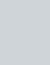Where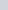is the resistivity of the material of the conductor.

It is directly proportional to the temperature of the conductor, it is as follow: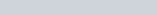Whereis the temperature coefficient of resistance.

Resistance and resistivity

## RESISTIVITY : BASIC

Resistivity is special type of resistance so it is also called specific resistance. It is resistance caused by any conductor having unit length and unit cross sectional area. The formula which represents the Resistivity is-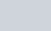Resistivity is also depends on the many factors such as :

• Cross sectional area of the conductor.
• Length of the conductor
• Temperature of the conductor
• Nature of the material of the conductor.

It’s SI unit is ohm-metre and it is denoted as Ω.m

## DIFFERENCE BETWEEN RESISTANCE AND RESISTIVITY

In this table you will see the difference between resistance and resistivity organised way.